# Javascript Function: Refactoring & Recursive

by Ridwan Fadilah, updated on Apr 14, 2020In the previous tutorial, we have introduced and saw some examples of how to use functions in javascript. Now, we'll learn more about javascript function and try to make the code writing more efficient.
Before that, I'll tell you, in this tutorial we just learning the basics of function with some examples that use often.

## Refactoring

Refactoring is a process to change the code to make it more 'good' without changing the functionality.

### Why refactoring?

The reason why we use refactoring is like this :

• Readability - Because a 'good' program is a program that is easy to read. Not only for the person who made it but also for the other people who are in a team.
• DRY (Do not Repeat Yourself) - No need to rewrite the code of a program.
• Testability - Code writing that will make it easier when testing.
• Performance - Code writing that upgradable performances.
• Maintainability - Easily managed and developed.

In the previous tutorial, we have an example of a javascript function to get a total sum. We'll use that and make a simple example of how to refactoring the code.

This is an example:

``````    function myFunction(a, b) {
let total;
total = a + b;

}

This function will return to value 22. If you see that, actually we can use a more efficient way. How to?

Just remove this :

``    let total;``

and this :

``    total = a + b;``

from the function body, then places the "a + b" to the return total:

``    return total = a + b;``

Now the code is more efficient than before:

``````    function myFunction(a, b) {
}

Let's try the code:

``````    <!DOCTYPE html>
<html lang="en">

<title>Function</title>

<body>

<script>
function myFunction(a, b) {
}

</script>

</body>

</html>``````

The function is still running well and keep produced the same value as before :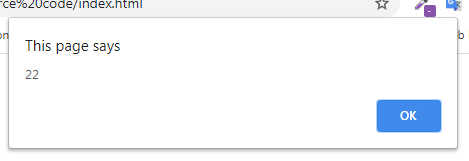The example above can we refactoring again:

``````    function myFunction(a, b) {
return a + b;
}

Example:

``````    <!DOCTYPE html>
<html lang="en">

<title>Function</title>

<body>

<script>
function myFunction(a, b) {
return a + b;
}

</script>

</body>

</html>``````

Same as before, the function is still running well :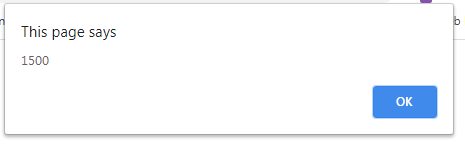That's the basis of refactoring function on javascript.

## Recursive

Recursive is a function that can call himself. Recursive must end with a condition. The end condition of recursive is called "Base Case"

If the function doesn't have the end condition or returning a value, then the function will continuously call himself and that will give an error message.

If the return value not set, it only stops the function (the function will not re-execute).

Example:

``````    <!DOCTYPE html>
<html lang="en">

<title>Function</title>

<body>

<script>
function test() {
return test();
}

test();
</script>

</body>

</html>``````

The code above will produce an error like this: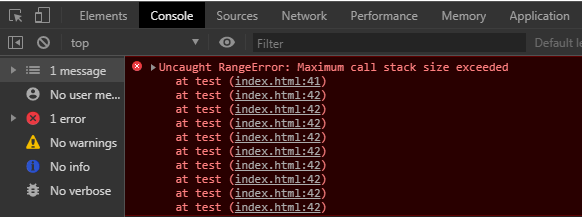It means too many recursive function calls.

For another example, I want to do countdown by the recursive function.

Example:

``````    <!DOCTYPE html>
<html lang="en">

<title>Function</title>

<body>

<script>
function countdown(n) {
console.log(n);

return countdown(n-1);
}

countdown(10);
</script>

</body>

</html>``````

The countdown is continuously called until it reaches the maximum calling limit :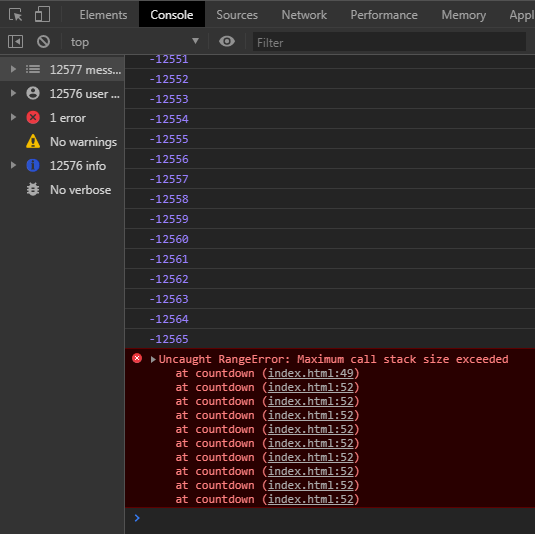If we want to stop the function, then we need to add a base case :

``````    if( n === 0 ) {
return;
}``````

With this, the countdown will stop when the "n" is reaches or the same as 0 (zero).

Example:

``````    <!DOCTYPE html>
<html lang="en">

<title>Function</title>

<body>

<script>
function countdown(n) {
if (n === 0) {
return;
}
console.log(n);

return countdown(n - 1);
}

countdown(10);
</script>

</body>

</html>``````

Now, recursive has stopped :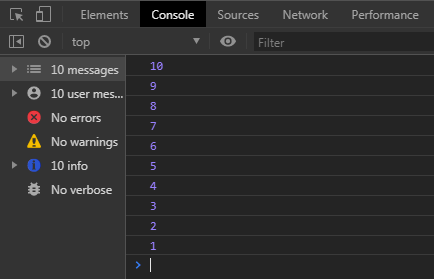All of the loopings can be made into a recursive, but recursive can't be made into a looping.

Here's a looping example:

``````    function countdown(n) {
for (var i = n; i >= 1; i--) {
console.log(i);
}
}

countdown(10);``````

That's some an example of recursive. For implementing recursive you can see the implementation below :

• Replacing the looping
• Fibonacci
• Find the structure of data list and tree
• Programming languages that not have a looping like Haskell, Prolog, Erlang, etc

Overall that's just basic, you can find and try the full source code of these examples on our GitHub.

That just the basic. If you need more deep learning about HTML, CSS, Javascript or related you can take the following cheap course:

• HTML & HTML5 For Beginners (A Practical Guide)• Web - HTML• Learn HTML, CSS, JAVASCRIPT• JavaScript:• Learn JavaScript Fundamentals• Learning JavaScript Shell ScriptingThanks!